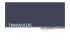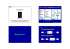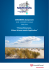# Characterization of a porous plate saturated with water

## Transcription

Characterization of a porous plate saturated with water
```Sound Intensity and Inverse Methods in Acoustics: Paper
ICA2016-534
Characterization of a porous plate saturated with water
using Bayesian inversion
Matti Niskanen(a) , Aroune Duclos(b) , Timo Lähivaara(c) , Olivier Dazel(d) ,
Jean-Philippe Groby(e) , Tomi Huttunen( f )
(a) Université
du Maine, France, [email protected]
du Maine, France, [email protected]
(c) University of Eastern Finland, Finland, [email protected]
(d) Université du Maine, France, [email protected]
(e) Université du Maine, France, [email protected]
(f) University of Eastern Finland, Finland, [email protected]
(b) Université
Abstract
The aim of this work is to estimate the properties of a poroelastic plate saturated by water. The
estimated properties are porosity, tortuosity, viscous characteristic length, flow resistivity, solid
density, bulk moduli of both the solid phase and the skeleton, and shear modulus. The characterization method consists of measuring the plate’s reflection and transmission coefficients y =
[R T ]> and then solving the inverse problem y = H(xx) + v , where H(xx) is the forward model, x
contains the parameters of interest and v is noise. The forward model uses the alternative 1962
Biot’s formulation. Wave equations derived from this formulation are solved using the state vector
formalism. Measurements are performed at normal incidence in a water tank in the ultrasonic
regime. The sample is a ceramic QF-20 from the Filtros Company. Transmission coefficient
is measured with two transducers while the reflection coefficient is measured with one transducer operating in a pulse-echo mode. In this work, the inverse problem is solved in the Bayesian
framework which is well-suited to handle measurement and model uncertainties. All the unknown
quantities are modeled as random variables. Furthermore, the prior models can be formulated
so that they carry information of the target. Results of the characterization process agree with
values found from the literature.
Keywords: porous materials, Biot theory, Bayesian inversion
Characterization of a porous plate saturated with
water using Bayesian inversion
1
Introduction
Characterizing the acoustic properties of porous materials is motivated by their use for numerous applications. These include designing better sound absorbing materials , estimating the
properties of cancellous bone for diagnosing osteoporosis  and monitoring groundwater reserves . To model the wave propagation accurately, elasticity of the solid part must be taken
into account. This is usually done using the Biot theory .
The goal of this work is to recover simultaneously all the parameters describing the acoustic
wave propagation in a poroelastic plate, namely the parameters involved in the Biot model,
using ultrasonic measurements. The modeled object is a homogeneous plate made of porous
ceramic that is used in filtration applications. The acoustic field is modeled assuming a onedimensional plane wave propagation at normal incidence. The measurements are performed
in water to better excite the elastic waves. Parameter inversion is carried out in the Bayesian
framework .
2
Theory
The characterizing process corresponds to solving an inverse problem. A forward problem is
included in the solution of the inverse one as an intermediate step. In the forward problem
wave propagation inside the poroelastic material is solved when its properties and boundary
conditions are known. In the inverse problem properties of the medium and geometry are
estimated so that the model fits observed data in the best possible way.
2.1 Forward model
Stress-strain relations after Biot’s 1962 formulation  are
(
σi j = 2Nεi j + (λc θ − αMξ )δi j ,
p = M(ξ − αθ ) ,
where σ is the total stress tensor, p the fluid pressure, N the shear modulus, εi j = 12 (ui, j + u j,i )
the strain tensor (with u the solid displacement), λc = λ +α 2 M the closed porosity bulk modulus,
θ = ui,i , α an elastic coupling coefficient, M an elastic parameter and ξ = −wi,i the fluid/solid
U − u ), where φ is the porosity and U the fluid displacement).
relative displacement (with w = φ (U
These variables can be related to the more commonly used 1956 Biot coefficients P, Q and R
by
2
α = φ (Q + R)/R,
M = R/φ 2 ,
λc = P − Q2 /R − 2N + a2 M ,
(1)
which can in turn be related to the bulk moduli of the fluid phase K f , solid phase Ks and
skeleton (solid less pore volume) Kb as 
Kb
Ks
)Ks + φ Kb
Ks
Kf
4
P=
+ N,
Kb
Ks
3
1−φ −
+φ
Ks
Kf
Kb
(1 − φ − φ Ks )
Ks
Q=
,
Kb
Ks
1−φ −
+φ
Ks
Kf
(1 − φ )(1 − φ −
R=
φ 2 Ks
.
Kb
Ks
1−φ −
+φ
Ks
Kf
Conservation of momentum and Darcy’s law give in the frequency domain:
(
ω 2 ρ f wi + ω 2 ρui = −σi j, j ,
ω 2 ρ f ui + ω 2 ρ̃eq wi = p, j ,
where ρ f is the density of the fluid, ρ = (1 − φ )ρs + φ ρ f is the bulk density of the medium (with
ρs density of the solid), and ρ̃eq is the mass parameter, defined as
ρ̃eq =
i
ρ f α∞ h
ωc
1 + i F(ω) .
φ
ω
(2)
The function F(ω) accounting for viscous losses was introduced in Ref.  as
s
F(ω) =
1 − iηρ f ω
2α∞
φ Λσ
2
,
(3)
where ωc = σ φ /(ρ f α∞ ) is the Biot frequency, σ the flow resistivity, Λ the viscous characteristic
length and η the fluid viscosity.
b = [σ
b22 , pb, ub2 , vb2 ] is first introduced, where the subscript 2 denotes the x2
The state vector W
component (normal to the plate), see Figure 1. The Biot equations can be rewritten in the form
(for more details see ):
3
Figure 1: A view of the configuration.
∂ b
b =0,
W − AW
∂ x2
⇒
b (L) = M W
b (0) ,
W
(4)
where M = eA L is a matrix relating the state vector at x2 = 0 to the state vector at x2 = L.
Assuming a plane incident wave at normal incidence the pressure fields left and right to the
plate (p and p ) can be written as
p = e−ik(x2 −L) + Reik(x2 −L) ,
(5)
p = Te−ikx2 .
After applying the boundary conditions one can solve the reflection and transmission coefficients are calculated from the following linear system

R
ub2 (L)

M L0 
−M
 T  = −SS ,
ub2 (0)

L
L
(6)
where
−1

1
LL = 
 0

k
ωρ f
where k = ω

0
0
,
1
0
−1

1
L0 = 
 0
− ωρk f


0
0
,
1
0

−1
 1 

S=
 0 ,
− ωρk f

(7)
p
K f /ρ f is the wavevector.
2.2 Inversion
The inverse problem is solved in the Bayesian framework (see for example Ref. ), where
all the unknowns are modeled as random variables and the information on these variables is
coded into probability distributions. The advantages of Bayesian inversion include the possibility
4
to explicitly define prior information about the variables and that, in addition to estimates for the
parameters, one can obtain confidence intervals for those estimates.
Statistical inversion is based on the Bayes’ formula, which states that the posterior probability
density πpost (xx|yy) is proportional to the prior density πpr (xx) multiplied by the likelihood π(yy|xx):
πpost (xx|yy) ∝ π(yy|xx)πpr (xx) ,
(8)
where x = [Λ, α∞ , σ , φ , Kb , Ks , N, ρs , L] is the vector of the estimated parameters which includes
the material properties and the thickness of the plate, and y is the measurement data.
The system can be described with the following observation model
(9)
y = H(xx) + v ,
where y = [R T ]> includes the reflection and transmission coefficients, H is the forward model,
x includes all the parameters of interest and v is the measurement noise. The noise v is
assumed to be normally distributed with zero mean and covariance Γv , so v ∼ N (0, Γv ).
The likelihood is formed from the probability distribution of the measurement noise πv as:
1
> −1
π(yy|xx) = πv (yy − H(xx)) ∝ exp − (yy − H(xx)) Γv (yy − H(xx)) .
2
(10)
Final step in the solution of the inverse problem is to explore the posterior density to calculate estimates for the parameters and their uncertainties. An often-used point estimate is the
maximum a posteriori (MAP) estimate which is defined as a point in the posterior that has the
largest probability. To calculate the estimates for the parameter uncertainty, a Markov chain
Monte Carlo sampling method is used, implemented using the Metropolis-Hastings algorithm
 .
3
Experimental setup
Reflection and transmission coefficients of the examined sample are measured experimentally.
This is done in a water tank where the sample is submerged for enough time so that complete
saturation is achieved. The sample material used here is a porous ceramic QF-20 manufactured by Filtros Ltd.
The measurement setup is based on the spectral ratio technique , where two measurements are needed: one with the plate and one without (the reference measurement). Then,
Fourier transforms of those measurements are divided to obtain R and T as:
T=
F {ytplate }
F {ytref }
e
−ikw L
,
R=
F {yrplate }
F {yrref }
,
(11)
5
Signal
generator
Duplexer
Oscilloscope
Computer
Signal
generator
Duplexer
Oscilloscope
Computer
Anechoic
termination
Figure 2: Measurement setup for the reflection measurements. Left: Measurement with the
plate. Right: reference measurement.
where y denotes the recorded time-domain signal. Transmission coefficient is multiplied by
e−ikw L , where kw is the wavenumber in water and L the thickness of the plate, to account for
the difference in path length induced by inserting the plate. Reflection measurements are performed with the transducers in pulse-echo (PE) mode, where a duplexer periodically changes
the transducer mode from emitting to receiving. Transmission measurements are performed
using two transducers, one emitting and the other receiving.
The reference measurement for T is easy to obtain as the plate just needs to be removed. The
reference for reflection can be obtained from the air-water interface by pointing the transducer
up, or from a transmission measurement using two transducers. The former requires precise
position of the transducer for it to be normal to the interface. The latter is inaccurate in other
ways since the operating mode and possibly characteristics of the transducers are different. To
obtain a reliable reference yrref , one can use two transducers in PE mode facing each other,
as shown in Figure 2. In this configuration, the emitting and receiving characteristics of both
transducers are recorded. Now the reflection measurements must be also performed with both
transducers, one measuring while the other is facing an anechoic wall. Then the transducers
are carefully switched around for another measurement of the same position using the other
transducer. Finally these two measurements are summed to obtain yrplate .
4
Results
The measured and modeled coefficients are complex valued so they include information also on
the phase change. Hence the vector y − H(xx) in the likelihood consists of four parts: magnitude
and phase for both R and T . The measurement error covariance Γv is approximated as constant
for each part, with no correlation between values so it is a diagonal matrix. The magnitude of
each part of Γv is set at 20% of the part’s maximum measured value.
For the prior model, each variable is considered to be independently distributed from the others
with Gaussian distribution, so x ∼ N (µx , Γx ). Expected values are found from data by the plate’s
manufacturer (see Table 1) and standard deviations are taken to be 20% of the expected value.
6
Figure 3: Measurements and the MAP-estimate.
For L the standard deviation is set at 1% of the expected value because it was measured
accurately with a vernier caliper. The distributions are also truncated at values that are not
physically admissible, so that for example the porosity can never be less than 0 or above 1.
Markov chain Monte Carlo integration of equation (8) was carried out for the measured data.
Four chains were run in parallel each with a length of 100,000 iterations. Initial values for each
chain were drawn from the prior distribution. A MAP-estimate is obtained as the most probable
value found with the MCMC-integration and the uncertainty intervals are standard deviations of
all the samples.
Table 1 shows the results obtained with the presented method, as well as values given by the
manufacturer. Figure 3 shows the measured values of R and T as well as the corresponding
reconstructed curves with the estimated parameters. As it can be seen, reconstructed curves
follow the measured ones closely. However, peaks in the magnitude of the transmission coefficient are underestimated slightly.
7
Table 1: Estimated parameter values and values given by the manufacturer.
Λ (µm)
α∞
σ (Nsm−4 )
φ
Kb (GPa)
Ks (GPa)
N (GPa)
ρs (kgm−3 )
L (mm)
5
MAP ± std
27.1 ± 4.3
1.98 ± 0.22
77.1 ± 17.5 · 106
0.41 ± 0.04
12.0 ± 2.4 − i(0.5 ± 0.1)
32.9 ± 9.5
8.7 ± 2.2 − i(0.1 ± 0.03)
2840 ± 370
24.9 ± 0.06
Manufacturer
20.1
1.84
67.9 · 106
0.4 ± 0.05
9.5 − i0.3
36.6
7.6 − i0.1
2760
24.9
Conclusions
A method for characterization of poro-elastic plates using Bayesian inversion was proposed.
Preliminary results show that a good agreement was found between the measurement and the
curves reconstructed from the values obtained by inversion, see Figure 3. However, there are
some remarks that should be considered. First, the measurement noise was assumed to be
distributed normally with zero mean and the model does not account for different types of noise
or modeling error. This is a topic of future work. Second remark is that because the posterior was found to be multi-modal, it cannot be said with certainty that the true MAP estimate
has been found given the finite amount of iterations. Enhanced MCMC methods  have
been developed to work better than the standard Metropolis-Hastings algorithm in the case of
multi-modal target functions. This point is also related to the accuracy of the error estimates
since the likelihood is constructed with statistics of the noise. Third, the prior was constructed
from the manufacturer data using Gaussian distributions. Other types of measurements could
be used to give more prior information, possibly giving reasons to employ other types of prior
distributions as well.
Acknowledgements
MN, AD, OD and JPG gratefully acknowledge the support from the LMAc projects Decimap and
PavNat. JPG gratefully acknowledges the support of the ANR Project METAUDIBLE (ANR-13BS09-003) co-funded by ANR and FRAE. TL and TH gratefully acknowledge the support from
based upon work from COST Action DENORMS CA15125, supported by COST (European
Cooperation in Science and Technology).
8
References
 L. De Ryck et al., ”Acoustic wave propagation and internal fields in rigid frame macroscopically inhomogeneous porous media”, J. Appl. Phys. 102, 024910 (2007).
 J. L. Buchanan, R. P. Gilbert and M. Y. Ou, ”Recovery of the parameters of cancellous bone
by inversion of effective velocities, and transmission and reflection coefficients”, Inverse
Problems 27, 125006 (2011).
 T. Lähivaara, N. F. Dudley Ward, T. Huttunen, Z. Rawlinson and J. P. Kaipio, ”Estimation
of aquifer dimensions from passive seismic signals in the presence of material and source
uncertainties”, Geophys. J. Int. 200, 1662-1675 (2015).
 M. A. Biot, ”Mechanics of deformation and acoustic propagation in porous media”, J. Appl.
Phys. 33, 1482-1498 (1962).
 J. P. Kaipio and E. Somersalo, ”Statistical and Computational Inverse Problems”, SpringerVerlag, 2005.
 J.-F. Allard and N. Atalla, Propagation of sound in porous media: modelling sound absorbing
materials, 1-358, 2nd edition, John Wiley & Sons Ltd., Chichester, United-Kingdom, (2009).
 D. L. Johnson, J. Koplik and R. Dashen, ”Theory of dynamic permeability and tortuosity in
fluid-saturated porous media”, J. Fluid Mech. 176, 379-402 (1987).
 G. Gautier et al., ”Propagation of acoustic waves in a one-dimensional macroscopically
inhomogeneous poroelastic material”, J. Acoust. Soc. Am. 130, 1390-1398 (2011).
 N. Metropolis, A. W. Rosenbluth, M. N. Rosenbluth, A. H. Teller and E. Teller, ”Equation of
state calculations by fast computing machins”, J. Chem. Phys 21, 1087-1092 (1953).
 W. Hastings, ”Monte Carlo sampling using Markov chains and their applications”,
Biometrika 57, 97-109 (1970).
 P. He and J. Zheng, ”Acoustic dispersion and attenuation measurement using both transmitted and reflected pulses”, Ultrasonics 39, 27-32 (2001).
 B. Hu, K.-W. Tsui, ”Distributed evolutionary Monte Carlo for Bayesian computing”, Comput.
Stat. Data Anal. 54, 688-697 (2010).
9
```

### SERGIOS AGAPIOU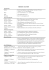### User Manual of how to inquire about Traffic Fines by the Mobile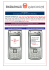### la teoria della deriva dei continenti e della tettonica a zolle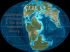### View - Aladdin Temp Rite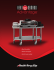### How I Engrave a Copper Plate### Inverse acoustic characterization of rigid frame porous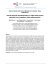### How to convert MTD Chipper/Shredder into Hammer Mill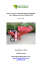### 2.2 mb - Mark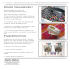### Star Plate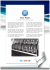### Axisymmetric wave propagation in multilayered poroelastic grounds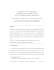### Frederic Weidling Thorsten Hohage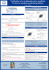### Alfred Wegener`s Theory of Continental Drift Became Modern Plate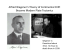### the Solutions for Narrow Web Printers Brochure### Inverting Beauty - ESMA European Society for Mathematics and the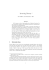### NUMERICAL CALCULATION OF DEFORMATION OF THREE DIMENSIONAL SAMPLE IN TRIAXIAL APPARATUS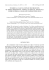### Inverse Functions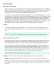### Proceedings of the 9th International Conference on Structural Dynamics, EURODYN... Porto, Portugal, 30 June - 2 July 2014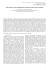### PDF hosted at the Radboud Repository of the Radboud University Nijmegen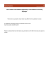### Zhdanov, M.S. and Yoshioka, K, 2003, Cross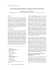### TRANSDUCERS